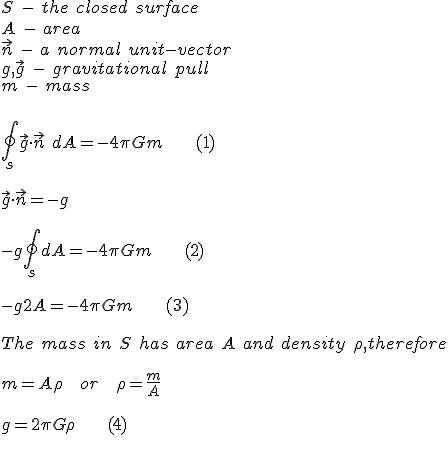Today we will be talking about how to use Gauss's Law to show that an infinite plane would have a finite gravitational pull. Now if you were to ask somebody how much gravitational pull an infinite amount of mass would exert, the common sense answer would be "infinite!" However, upon inspection we can see that certain configurations of mass would actually yield finite gravitational pulls.

This article requires an understanding of surface integrals and so likely the math will be beyond many. However, I will do my best to explain it in the simplest terms possible. If you come across some math you don't understand, do like I do and continue reading past it. You can later go back with what you learned and likely will have a better understanding about it.

First we will introduce a symbol that likely has hidden itself from many of our readers educations. This is to no shame though, I've met Ph.Ds who were not familiar with this! One astrophysicist, working on her PhD dissertation, went so far as to proclaim "Fake Math!" I have to admit, that one made me chuckle a bit to myself. It must be remembered that no one can possibly hope to know everything, especially when we seek to specialize.

This curious symbol looks like one you may have seen before, but it acts somewhat differently. You may be familiar with the integral, with which it shares many properties: ∫. Ignoring the terse mathematical definition, we will use a somewhat easy to understand one. Leibniz, its creator, thought of it as an infinite sum of infinitesimal summands. So, taking the integral over a small portion of a line, you can find the sum of its area above or below the curve by looking at the sum of infinitely small slices of it and adding it all up.

The symbol you may be less familiar with is that of the closed surface integral ∮. The difference here visually of course is the introduction of that circle in the middle. Think of it roughly as a generalization of the integral over a surface instead. So instead of infinitely small slices, you will be dealing with infinitely small surfaces!

The second key to the puzzle we will need to use to properly examine the gravitational affect of an infinite plane is Gauss's law. Usually used in electromagnetism, Gauss's law actually applies to gravitational forces as well since they both share an inverse square relationship 1/distance2 to their strength. It can be stated as follows:

gn dA = -4πGm

Lets say we have some mass m. We pretend to create a surface around the mass. We divide this surface up into infinitesimal parts, each with an area of dA. Remember that the integral is summing up an infinite amount of infinitesimal surfaces? We take the infinitesimal bits of the area (dA) and sum them over the entire closed surface. Each infinitesimal "bit" has an n. n is a unit vector that is perpendicular, so facing away from the surface at a right angle away. You might want to visualize that n represents "up" since we are dealing with a plane. g is the acceleration due to gravity, pointing towards the mass. In our case, this should be "down."

We can use this to examine the gravitational influences of any body. If we were looking at point mass, we would use a sphere. If we were looking at an infinite plane, as we are, we will use a pillbox for our surface.

When we look at the pillbox, we can simplify things nicely. We see the curved surface of the pillbox will "cancel out" its own gravitational influence and contribute nothing. A simple way to think of this is that each point on the cylinder is counterbalanced with another point on the cylinder. This coincidently also shows the infinite plane is a stable body as each point on the plane itself is also counter-balanced by the points around it horizontally, thus answering the common question "Why wouldn't mass form into a sphere?" A more accurate way would be to realize this is that g is at a right angle to our "up" ( n ) at all points; all points have an opposite point that is facing the opposite direction as well, and so g•n = 0.

We are almost there - this leaves us with only the circle caps to deal with.

Looking at the caps we realize that g and n are parallel and opposite each other and so we realize gn = -g. This leaves the surface integral of just the pillbox ends. Since we have 2 of them and the integral is the sum of the parts of dA, we have -g∮dA = g2A leaving our equation as: -g 2A = -4πGm. From there its easy to see g's value is finite - g= 2πGm / A. This is further realized by noting mass = (density * Area), giving us g A= 2πGpA, or g = 2πG p. This is clearly a finite value. If we wished we could continue from here to calculate the depth of the plane using the average density of Earth.

Given it also has depth we are looking at the case of m = (density * Area * depth). This gives us instead g = 2πG p d, where d is depth.

g = 9.81 m/s/s
G = 6.754×10−11 m3 kg−1 s−2
p = 5.51 g/cm³ , the average density of earth

Giving us d = g / (2πG p). This evaluates to around 4 195.43 kilometers deep, thus showing false my early hypothesis of 9000 km deep.